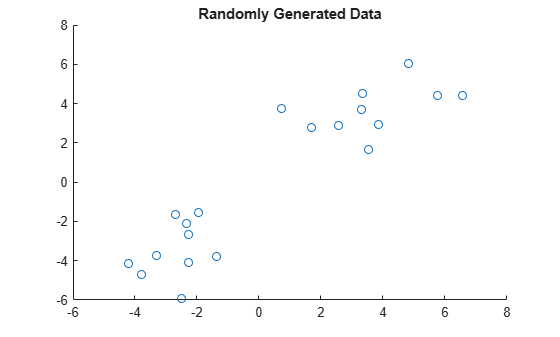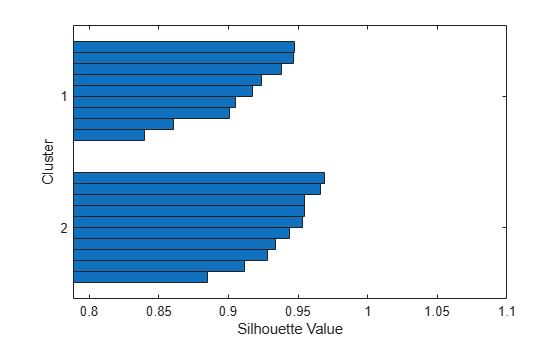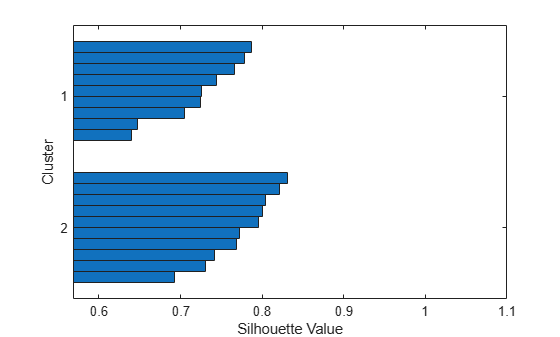# silhouette

Silhouette plot

## Syntax

``silhouette(X,clust)``
``silhouette(X,clust,Distance)``
``silhouette(X,clust,Distance,DistParameter)``
``s = silhouette(___)``
``[s,h] = silhouette(___)``

## Description

example

````silhouette(X,clust)` plots cluster silhouettes for the n-by-p input data matrix `X`, given the cluster assignment `clust` of each point (observation) in `X`.```

example

````silhouette(X,clust,Distance)` plots the silhouettes using the inter-point distance metric specified in `Distance`.```

example

````silhouette(X,clust,Distance,DistParameter)` accepts one or more additional distance metric parameter values when you specify `Distance` as a custom distance function handle `@distfun` that accepts the additional parameter values.```

example

````s = silhouette(___)` returns the silhouette values in `s` for any of the input argument combinations in the previous syntaxes without plotting the cluster silhouettes.```

example

````[s,h] = silhouette(___)` plots the silhouettes and returns the figure handle `h` in addition to the silhouette values in `s`.```

## Examples

collapse all

Create silhouette plots from clustered data using different distance metrics.

Generate random sample data.

```rng('default') % For reproducibility X = [randn(10,2)+3;randn(10,2)-3];```

Create a scatter plot of the data.

```scatter(X(:,1),X(:,2)); title('Randomly Generated Data');```The scatter plot shows that the data appears to be split into two clusters of equal size.

Partition the data into two clusters using `kmeans` with the default squared Euclidean distance metric.

`clust = kmeans(X,2);`

`clust` contains the cluster indices of the data.

Create a silhouette plot from the clustered data using the default squared Euclidean distance metric.

`silhouette(X,clust)`The silhouette plot shows that the data is split into two clusters of equal size. All the points in the two clusters have large silhouette values (0.8 or greater), indicating that the clusters are well separated.

Create a silhouette plot from the clustered data using the Euclidean distance metric.

`silhouette(X,clust,'Euclidean')`The silhouette plot shows that the data is split into two clusters of equal size. All the points in the two clusters have large silhouette values (0.6 or greater), indicating that the clusters are well separated.

Compute the silhouette values from clustered data.

Generate random sample data.

```rng('default') % For reproducibility X = [randn(10,2)+1;randn(10,2)-1];```

Cluster the data in `X` based on the sum of absolute differences in distance by using `kmeans`.

`clust = kmeans(X,2,'distance','cityblock');`

`clust` contains the cluster indices of the data.

Compute the silhouette values from the clustered data. Specify the distance metric as `'cityblock'` to indicate that the `kmeans` clustering is based on the sum of absolute differences.

`s = silhouette(X,clust,'cityblock')`
```s = 20×1 0.0816 0.5848 0.1906 0.2781 0.3954 0.4050 0.0897 0.5416 0.6203 0.6664 ⋮ ```

Find silhouette values from clustered data using a custom chi-square distance metric. Verify that the chi-square distance metric is equivalent to the Euclidean distance metric, but with an optional scaling parameter.

Generate random sample data.

```rng('default'); % For reproducibility X = [randn(10,2)+3;randn(10,2)-3];```

Cluster the data in `X` using `kmeans` with the default squared Euclidean distance metric.

`clust = kmeans(X,2);`

Find silhouette values and create a silhouette plot from the clustered data using the Euclidean distance metric.

`[s,h] = silhouette(X,clust,'Euclidean')````s = 20×1 0.6472 0.7241 0.5682 0.7658 0.7864 0.6397 0.7253 0.7783 0.7054 0.7442 ⋮ ```
```h = Figure (1) with properties: Number: 1 Name: '' Color: [1 1 1] Position: [348 480 583 437] Units: 'pixels' Show all properties ```

The chi-square distance between `J`-dimensional points x and z is

`$\chi \left(x,z\right)=\sqrt{\sum _{j=1}^{J}{w}_{j}{\left({x}_{j}-{z}_{j}\right)}^{2}},$`

where ${w}_{j}$ is the weight associated with dimension j.

Set weights for each dimension and specify the chi-square distance function. The distance function must:

• Take as input arguments the n-by-p input data matrix `X`, one row of `X` (for example, `x`), and a scaling (or weight) parameter `w`.

• Calculate the distance from `x` to each row of `X`.

• Return a vector of length n. Each element of the vector is the distance between the observation corresponding to `x` and the observations corresponding to each row of `X`.

```w = [0.4; 0.6]; % Set arbitrary weights for illustration chiSqrDist = @(x,Z,w)sqrt((bsxfun(@minus,x,Z).^2)*w);```

Find silhouette values from the clustered data using the custom distance metric `chiSqrDist`.

`s1 = silhouette(X,clust,chiSqrDist,w)`
```s1 = 20×1 0.6288 0.7239 0.6244 0.7696 0.7957 0.6688 0.7386 0.7865 0.7223 0.7572 ⋮ ```

Set the weight for both dimensions to 1 to use `chiSqrDist` as the Euclidean distance metric. Find silhouette values and verify that they are the same as the values in `s`.

```w2 = [1; 1]; s2 = silhouette(X,clust,chiSqrDist,w2); AreValuesEqual = isequal(s2,s)```
```AreValuesEqual = logical 1 ```

The silhouette values are the same in `s` and `s2`.

## Input Arguments

collapse all

Input data, specified as a numeric matrix of size n-by-p. Rows correspond to points, and columns correspond to coordinates.

Data Types: `single` | `double`

Cluster assignment, specified as a categorical variable, numeric vector, character matrix, string array, or cell array of character vectors containing a cluster name for each point in `X`.

`silhouette` treats `NaN`s and empty values in `clust` as missing values and ignores the corresponding rows of `X`.

Data Types: `single` | `double` | `char` | `string` | `cell` | `categorical`

Distance metric, specified as a character vector, string scalar, or function handle, as described in this table.

MetricDescription
`'Euclidean'`

Euclidean distance

`'sqEuclidean'`

Squared Euclidean distance (default)

`'cityblock'`

Sum of absolute differences

`'cosine'`

One minus the cosine of the included angle between points (treated as vectors)

`'correlation'`

One minus the sample correlation between points (treated as sequences of values)

`'Hamming'`

Percentage of coordinates that differ

`'Jaccard'`

Percentage of nonzero coordinates that differ

VectorA numeric row vector of pairwise distances, in the form created by the `pdist` function. `X` is not used in this case, and can safely be set to `[]`.
`@distfun`

Custom distance function handle. A distance function has the form

```function D = distfun(X0,X,`DistParameter`) % calculation of distance ...```
where

• `X0` is a `1`-by-p vector containing a single point (observation) of the input data matrix `X`.

• `X` is an n-by-p matrix of points.

• `DistParameter` represents one or more additional parameter values specific to `@distfun`.

• `D` is an n-by-`1` vector of distances, and `D(k)` is the distance between observations `X0` and `X(k,:)`.

Example: `'cosine'`

Data Types: `char` | `string` | `function_handle` | `single` | `double`

Distance metric parameter value, specified as a positive scalar, numeric vector, or numeric matrix. This argument is valid only when you specify a custom distance function handle `@distfun` that accepts one or more parameter values in addition to the input parameters `X0` and `X`.

Example: `silhouette(X,clust,distfun,p1,p2)` where `p1` and `p2` are additional distance metric parameter values for `@distfun`

Data Types: `single` | `double`

## Output Arguments

collapse all

Silhouette values, returned as an n-by-`1` vector of values ranging from `–1` to `1`. A silhouette value measures how similar a point is to points in its own cluster, when compared to points in other clusters. Values range from `–1` to `1`. A high silhouette value indicates that a point is well matched to its own cluster, and poorly matched to other clusters.

Data Types: `single` | `double`

Figure handle, returned as a scalar. You can use the figure handle to query and modify figure properties. For more information, see Figure Properties.

collapse all

### Silhouette Value

The silhouette value for each point is a measure of how similar that point is to other points in the same cluster, compared to points in other clusters.

The silhouette value si for the ith point is defined as

`${s}_{i}=\frac{\left({b}_{i}-{a}_{i}\right)}{\mathrm{max}\left({a}_{i},{b}_{i}\right)},$`

where ai is the average distance from the ith point to the other points in the same cluster as i, and bi is the minimum average distance from the ith point to points in a different cluster, minimized over the clusters. If the ith point is the only point in its cluster, then the silhouette value si is set to 1.

The silhouette values range from –1 to 1. A high silhouette value indicates that the point is well matched to its own cluster, and poorly matched to other clusters. If most points have a high silhouette value, then the clustering solution is appropriate. If many points have a low or negative silhouette value, then the clustering solution might have too many or too few clusters. You can use silhouette values as a clustering evaluation criterion with any distance metric.

 Kaufman L., and P. J. Rousseeuw. Finding Groups in Data: An Introduction to Cluster Analysis. Hoboken, NJ: John Wiley & Sons, Inc., 1990.